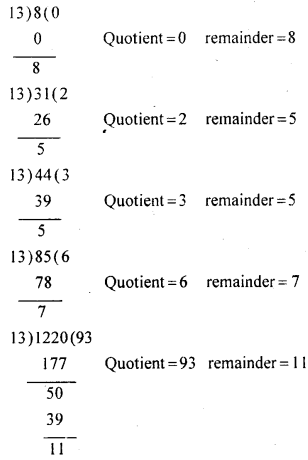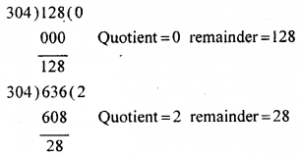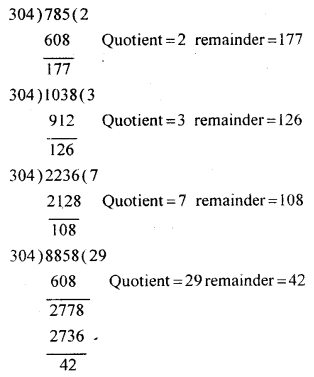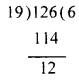# KSEEB Solutions for Class 8 Maths Chapter 1 Playing with Numbers Ex 1.3

In this chapter, we provide KSEEB SSLC Class 8 Maths Chapter 1 Playing with Numbers Ex 1.3 for English medium students, Which will very helpful for every student in their exams. Students can download the latest KSEEB SSLC Class 8 Maths Chapter 1 Playing with Numbers Ex 1.3 pdf, free KSEEB SSLC Class 8 Maths Chapter 1 Playing with Numbers Ex 1.3 pdf download. Now you will get step by step solution to each question.

## Karnataka Board Class 8 Maths Chapter 1 Playing with Numbers Ex 1.3

Question 1.
Find the quotient and remainder when each of the following number is divided by 13 : 8, 31, 44, 85, 1220.Question 2.
Find the quotient and the remainder when each of the following numbers are divided by 304.
128, 636, 785, 1038, 2236, 8858Question 3.
Find the least natural number larger than 100 which leaves the remainder 12 when divided by 19.
The least number greater than 100 divisible by 19 is 114.
Adding 12 to this number we get 114 + 12 = 126.
126 leaves 12 as the remainder when divided by 19.Question 4.
What is the least natural number you have to add to 1024 to get a multiple of 181?
The Multiples of 181 are 181, 362, 543, 724, 905, 1086.
The nearest multiple of 181 to 1024 is 1.086.
Hence 62 (1086 – 1024) must be added to 1024 to get a multiple of 181.

All Chapter KSEEB Solutions For Class 8 maths

—————————————————————————–

All Subject KSEEB Solutions For Class 9

*************************************************

I think you got complete solutions for this chapter. If You have any queries regarding this chapter, please comment on the below section our subject teacher will answer you. We tried our best to give complete solutions so you got good marks in your exam.

If these solutions have helped you, you can also share kseebsolutionsfor.com to your friends.

Best of Luck!!